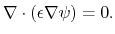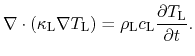## 4.1.7 Insulator Equations

In insulating materials only the Poisson equation (4.11) and the lattice heat flow equation (4.22) are solved. The carrier continuity equations are not solved because doping and mobile carriers are neglected. This reduces the Poisson equation to the Laplace equation:(4.26)

The heat generation term in (4.22) is also neglected:(4.27)

As a result neither carriers nor current are considered in insulators, however the consideration of charges at semiconductor/insulator interfaces is unaffected.

S. Vitanov: Simulation of High Electron Mobility Transistors